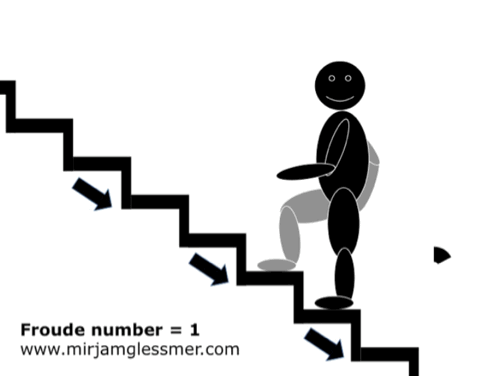# My escalator analogy for Froude numbers

The Froude number Fr=u/c is the ratio of a typical velocity of a current (u) and the phase velocity of the typical waves in that area (c). It thus describes whether a flow is subcritical for Fr<1 (i.e. with a current that is slower than the waves, so waves can propagate in any direction), or supercritical for Fr>1 (the current is moving faster than the waves, so waves can’t propagate upstream and are washed downstream with the current), and the transition between the two, where a hydraulic jump occurs (Fr=1).

Since many people find this hard to imagine, I like to use the “escalator analogy” (and please don’t try this at home or wherever you find the next elevator). But I think it works rather well as an analogy.

For Fr<1, the person on the elevator is walking faster than the elevator is moving. The person can thus get to where it wants to get, even though the elevator is running the opposite direction.

At Fr=1, the escalator moves as fast as the person, so the person is stuck in place.

And then for Fr>1, the escalator is moving faster than the person is, so the person gets “washed downstream” with the elevator.

What do you think? Is this analogy working for you? If yes, I might spend some more time on the animations. If no, well, I still had fun :-)